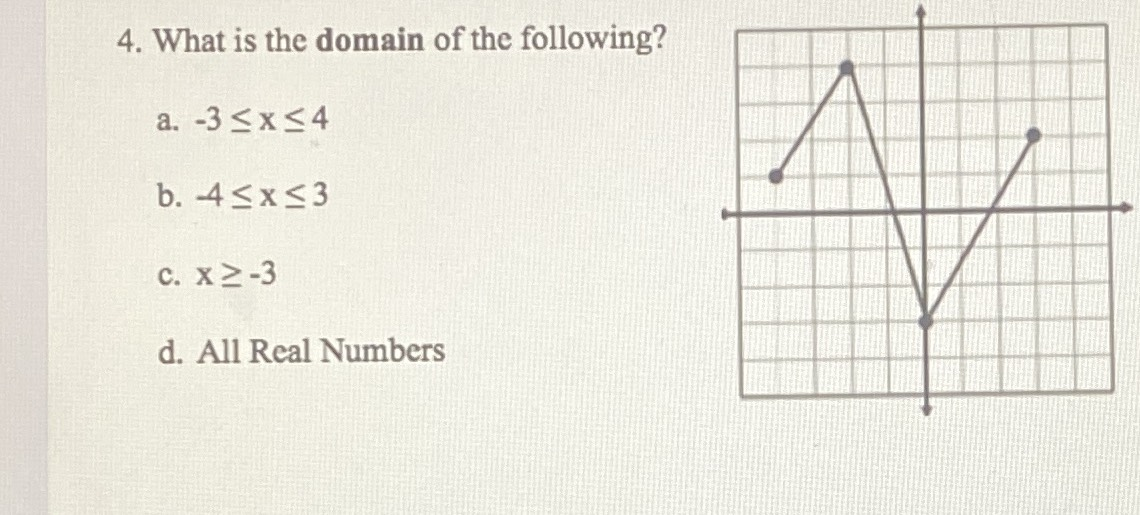### ¿Todavía tienes preguntas de matemáticas?

Pregunte a nuestros tutores expertos
Algebra
Pregunta4. What is the domain of the following?

a. $$- 3 \leq x \leq 4$$

b. $$- 4 \leq x \leq 3$$

c. $$x \geq - 3$$

d. All Real Numbers